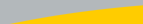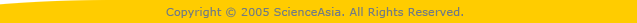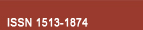| Home  | About ScienceAsia  | Publication charge  | Advertise with us  | Subscription for printed version  | Contact us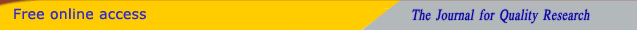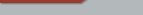Editorial Board Journal Policy Instructions for Authors Online submission Author Login Reviewer Login Volume 48 Number 5 Volume 48 Number 4 Volume 48 Number 3 Volume 48 Number 2 Volume 48S Number 1 Volume 48 Number 1 Earlier issuesVolume 47 Number 6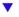Volume 48 Number 1previous article next articleResearch articles

ScienceAsia 48 (2022): 107-114 |doi: 10.2306/scienceasia1513-1874.2022.027

# Meromorphic solutions of some types of q-difference differential equation and delay differential equation

## Minfeng Chena,*, Zongsheng Gaob, Jilong Zhangb

ABSTRACT:     In this paper, we investigate the existence of rational solutions and value distribution of non-rational meromorphic solutions with the finite order of delay differential equation w(z + 1) ? w(z ? 1) + a w 0 (z) w(z) = b, where a ? C{0}, b ? C are constants. In addition, necessary conditions are obtained for q-difference differential equation w(qz) ? w(z/q) + a(z) w 0 (z) w(z) = R(z, w(z)) to admit a non-rational meromorphic solution of zero-order, where |q| ∈ {0, 1}, a(z) is rational and R(z, w(z)) is rational in w(z) with rational coefficients in z.